Скачать презентацию Knowledge representation 1 The importance of knowledge

02366a75e424b9c5c7fa2395271f0164.ppt

• Количество слайдов: 72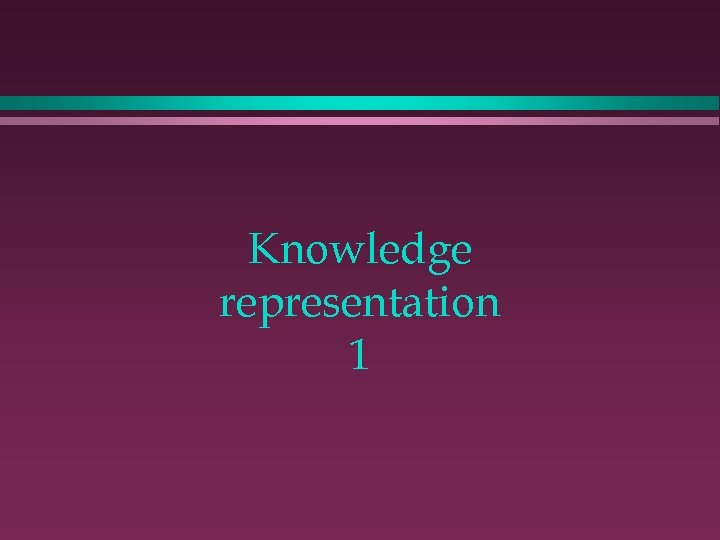Knowledge representation 1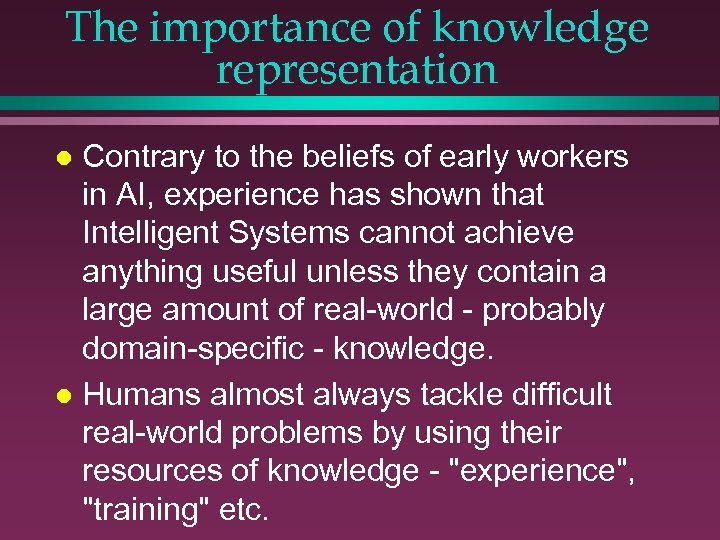The importance of knowledge representation Contrary to the beliefs of early workers in AI, experience has shown that Intelligent Systems cannot achieve anything useful unless they contain a large amount of real-world - probably domain-specific - knowledge. l Humans almost always tackle difficult real-world problems by using their resources of knowledge - "experience", "training" etc. l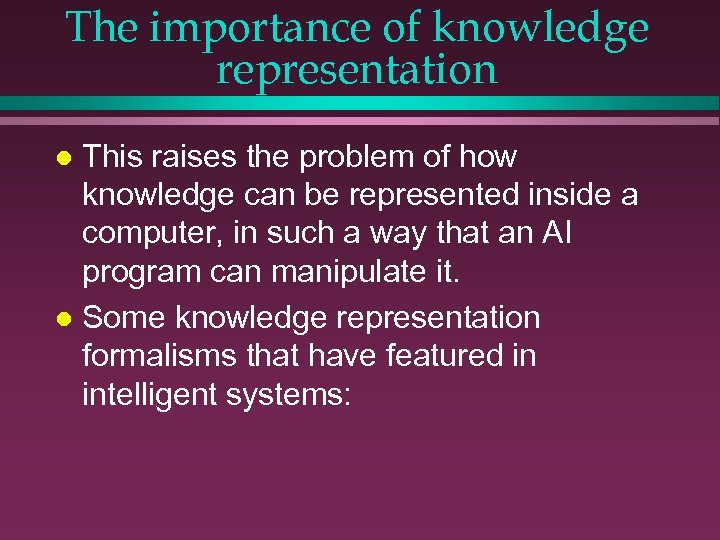The importance of knowledge representation This raises the problem of how knowledge can be represented inside a computer, in such a way that an AI program can manipulate it. l Some knowledge representation formalisms that have featured in intelligent systems: l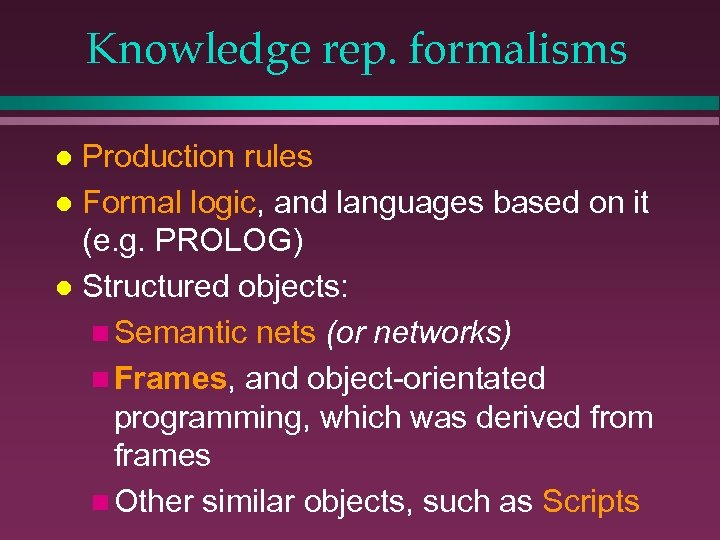Knowledge rep. formalisms Production rules l Formal logic, and languages based on it (e. g. PROLOG) l Structured objects: n Semantic nets (or networks) n Frames, and object-orientated programming, which was derived from frames n Other similar objects, such as Scripts l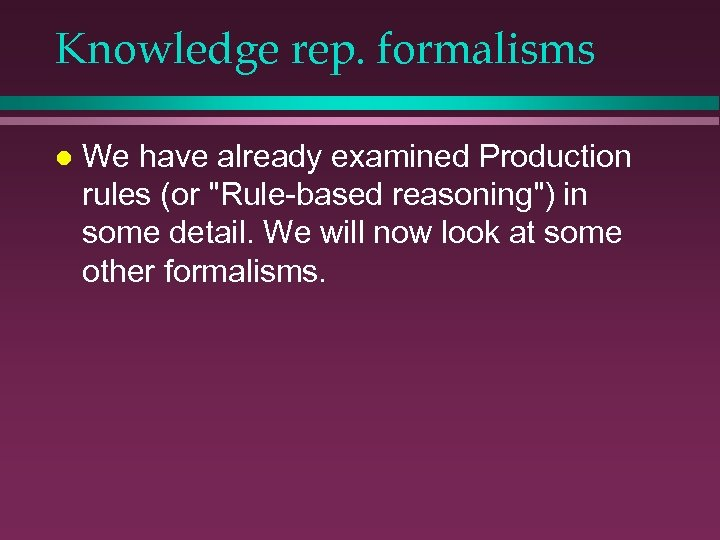Knowledge rep. formalisms l We have already examined Production rules (or "Rule-based reasoning") in some detail. We will now look at some other formalisms.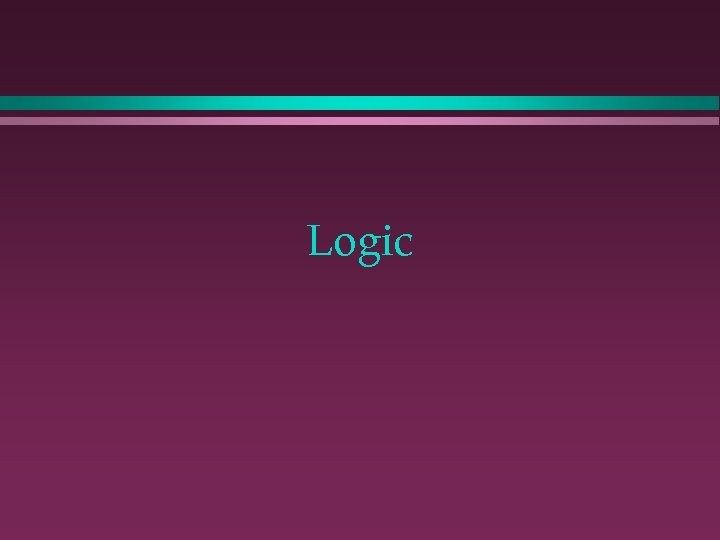Logic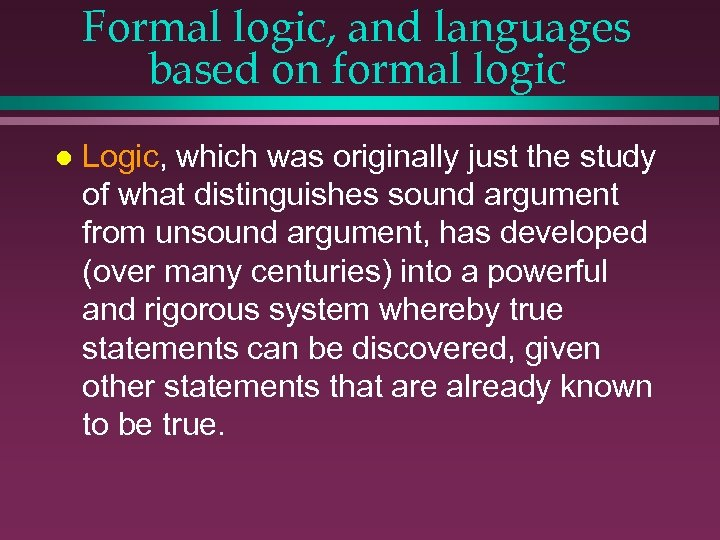Formal logic, and languages based on formal logic l Logic, which was originally just the study of what distinguishes sound argument from unsound argument, has developed (over many centuries) into a powerful and rigorous system whereby true statements can be discovered, given other statements that are already known to be true.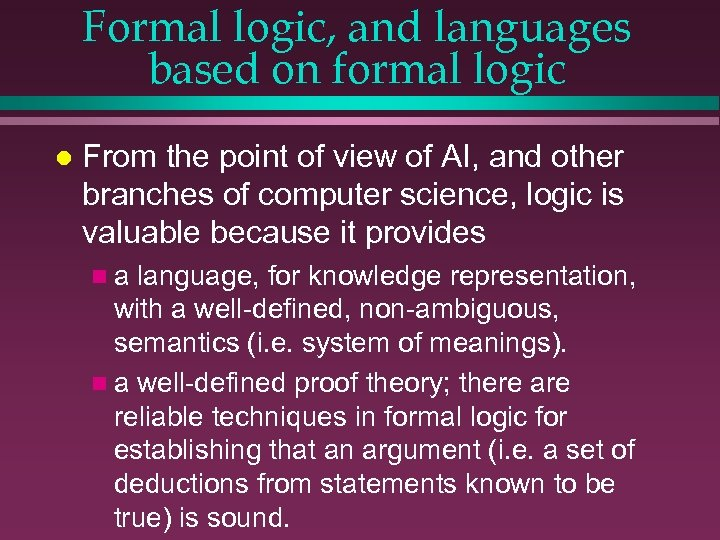Formal logic, and languages based on formal logic l From the point of view of AI, and other branches of computer science, logic is valuable because it provides na language, for knowledge representation, with a well-defined, non-ambiguous, semantics (i. e. system of meanings). n a well-defined proof theory; there are reliable techniques in formal logic for establishing that an argument (i. e. a set of deductions from statements known to be true) is sound.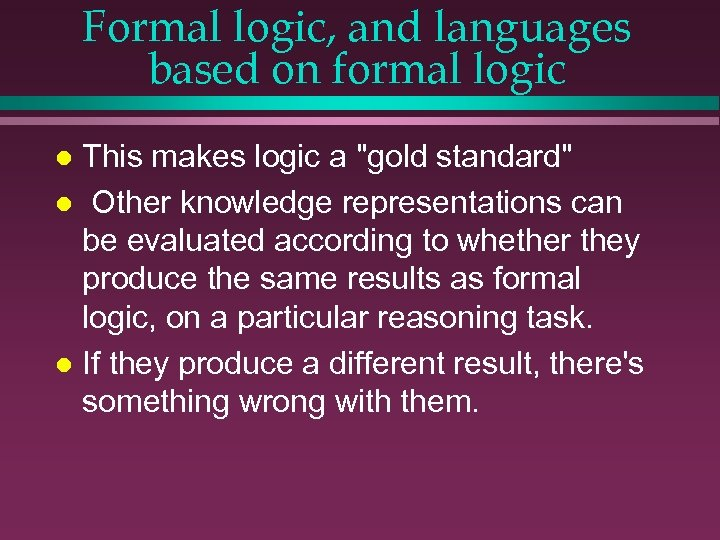Formal logic, and languages based on formal logic This makes logic a "gold standard" l Other knowledge representations can be evaluated according to whether they produce the same results as formal logic, on a particular reasoning task. l If they produce a different result, there's something wrong with them. l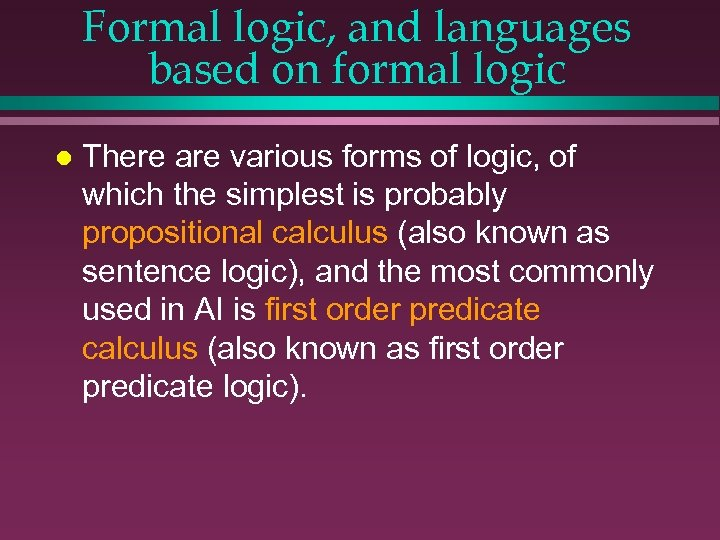Formal logic, and languages based on formal logic l There are various forms of logic, of which the simplest is probably propositional calculus (also known as sentence logic), and the most commonly used in AI is first order predicate calculus (also known as first order predicate logic).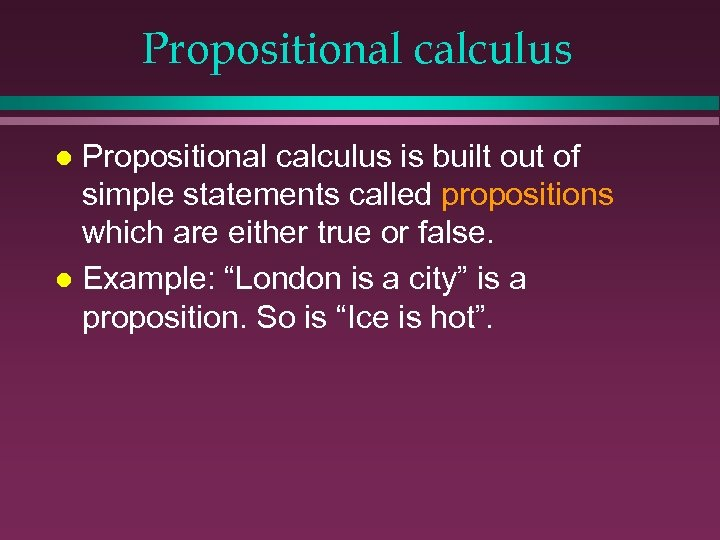Propositional calculus is built out of simple statements called propositions which are either true or false. l Example: “London is a city” is a proposition. So is “Ice is hot”. l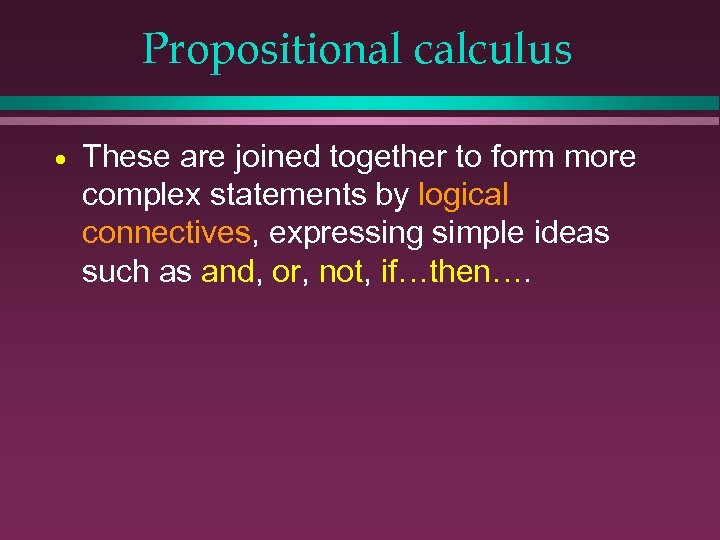Propositional calculus · These are joined together to form more complex statements by logical connectives, expressing simple ideas such as and, or, not, if…then….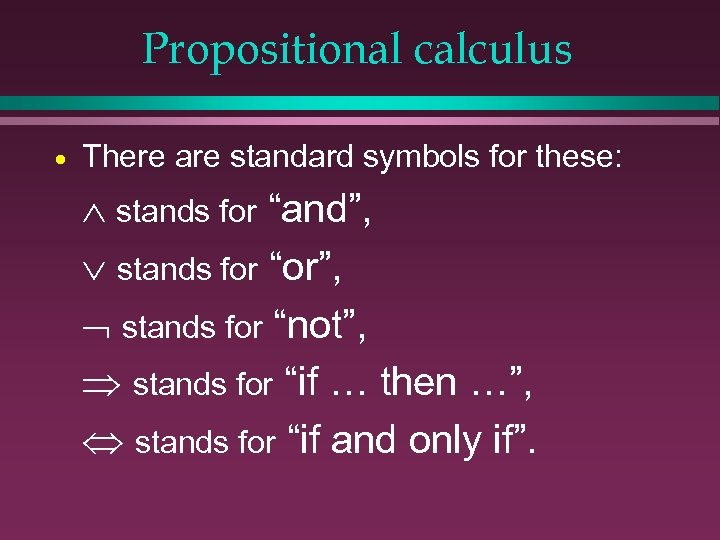Propositional calculus · There are standard symbols for these: stands for “and”, stands for “or”, Ø stands for “not”, stands for “if … then …”, Û stands for “if and only if”.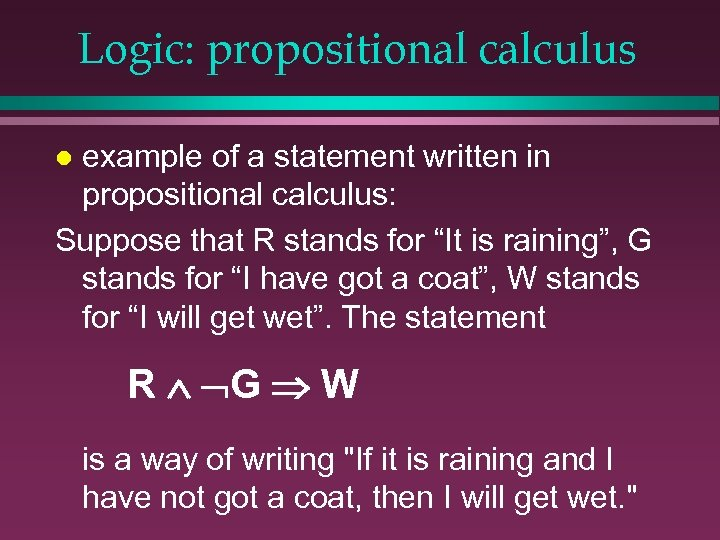Logic: propositional calculus example of a statement written in propositional calculus: Suppose that R stands for “It is raining”, G stands for “I have got a coat”, W stands for “I will get wet”. The statement l R Ù ØG Þ W is a way of writing "If it is raining and I have not got a coat, then I will get wet. "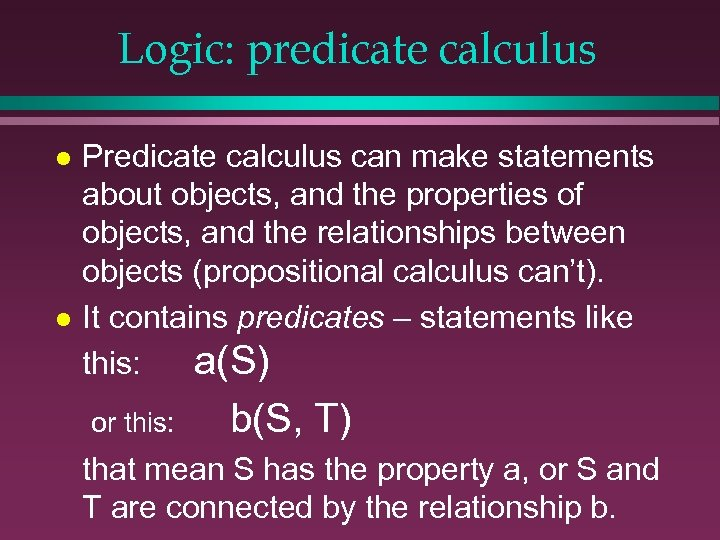Logic: predicate calculus l l Predicate calculus can make statements about objects, and the properties of objects, and the relationships between objects (propositional calculus can’t). It contains predicates – statements like this: a(S) or this: b(S, T) that mean S has the property a, or S and T are connected by the relationship b.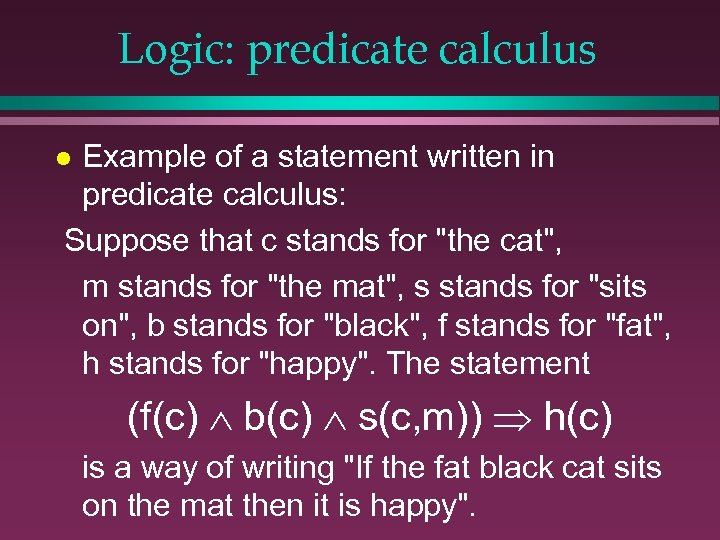Logic: predicate calculus Example of a statement written in predicate calculus: Suppose that c stands for "the cat", m stands for "the mat", s stands for "sits on", b stands for "black", f stands for "fat", h stands for "happy". The statement l (f(c) b(c) s(c, m)) h(c) is a way of writing "If the fat black cat sits on the mat then it is happy".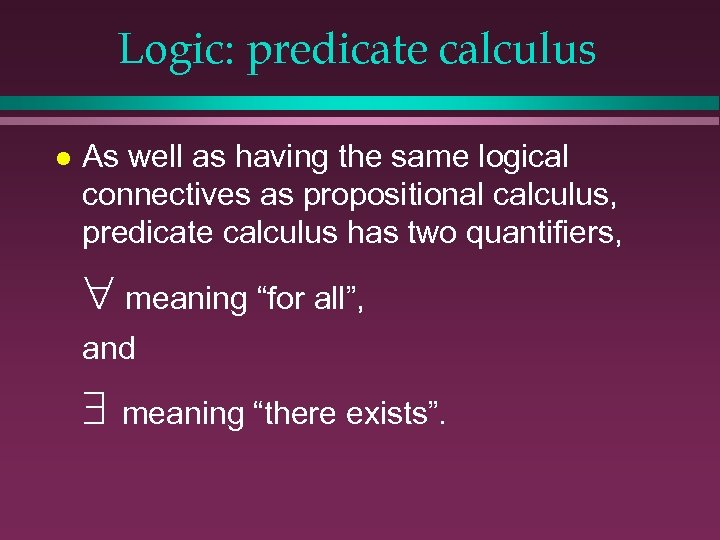Logic: predicate calculus l As well as having the same logical connectives as propositional calculus, predicate calculus has two quantifiers, meaning “for all”, and meaning “there exists”.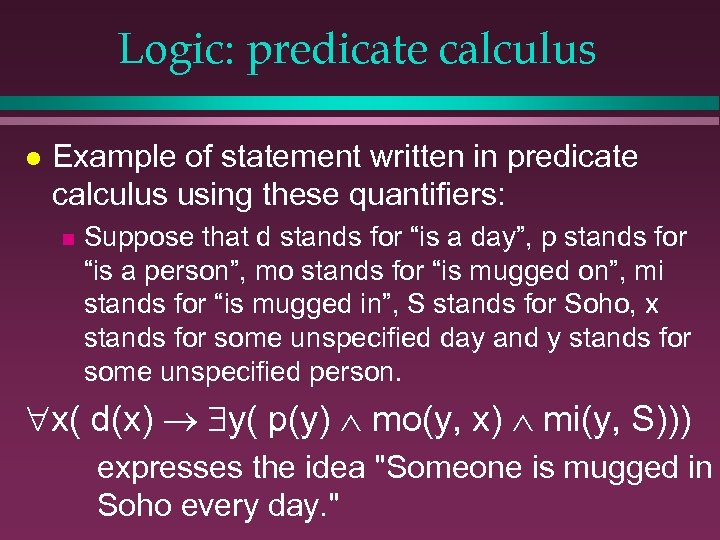Logic: predicate calculus l Example of statement written in predicate calculus using these quantifiers: n Suppose that d stands for “is a day”, p stands for “is a person”, mo stands for “is mugged on”, mi stands for “is mugged in”, S stands for Soho, x stands for some unspecified day and y stands for some unspecified person. x( d(x) y( p(y) mo(y, x) mi(y, S))) expresses the idea "Someone is mugged in Soho every day. "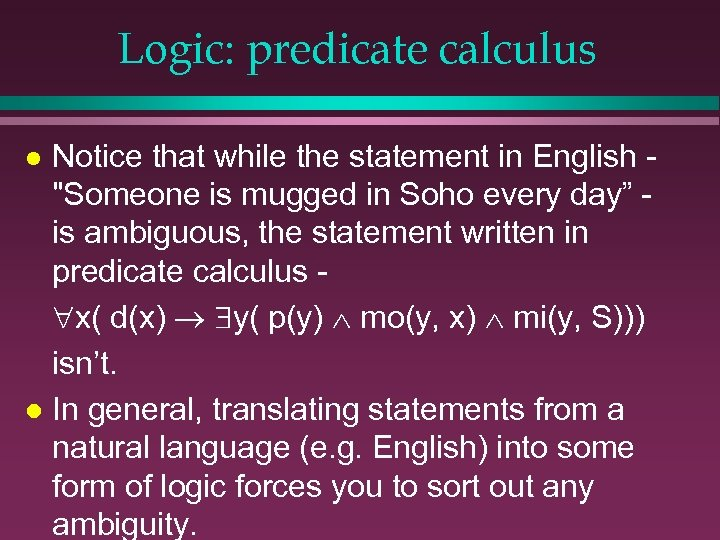Logic: predicate calculus Notice that while the statement in English "Someone is mugged in Soho every day” is ambiguous, the statement written in predicate calculus x( d(x) y( p(y) mo(y, x) mi(y, S))) isn’t. l In general, translating statements from a natural language (e. g. English) into some form of logic forces you to sort out any ambiguity. l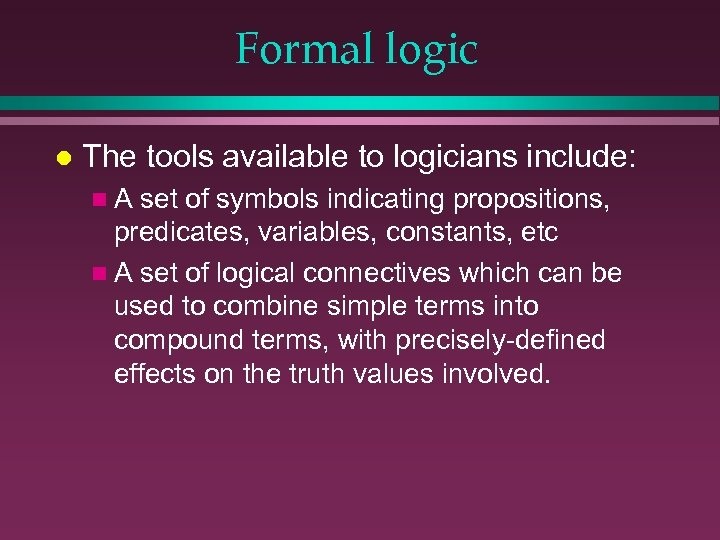Formal logic l The tools available to logicians include: n. A set of symbols indicating propositions, predicates, variables, constants, etc n A set of logical connectives which can be used to combine simple terms into compound terms, with precisely-defined effects on the truth values involved.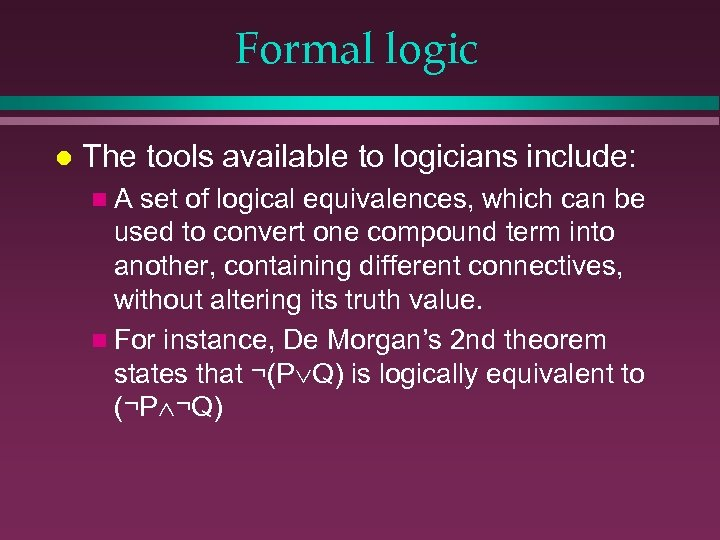Formal logic l The tools available to logicians include: n. A set of logical equivalences, which can be used to convert one compound term into another, containing different connectives, without altering its truth value. n For instance, De Morgan’s 2 nd theorem states that ¬(P Q) is logically equivalent to (¬P ¬Q)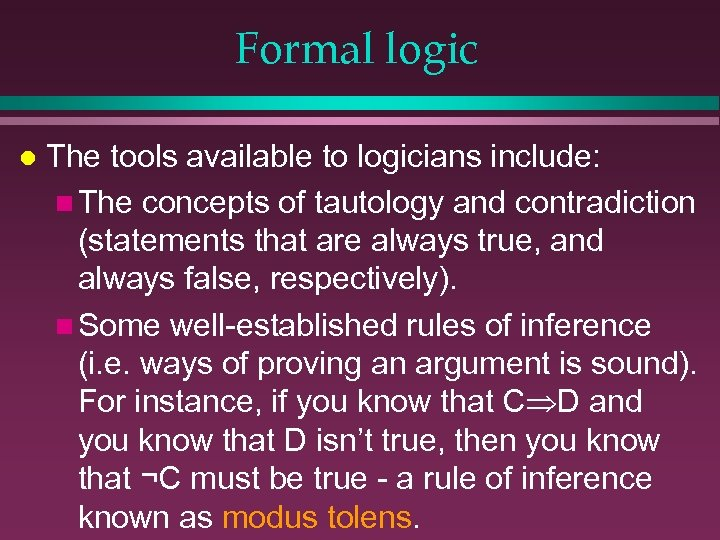Formal logic l The tools available to logicians include: n The concepts of tautology and contradiction (statements that are always true, and always false, respectively). n Some well-established rules of inference (i. e. ways of proving an argument is sound). For instance, if you know that C D and you know that D isn’t true, then you know that ¬C must be true - a rule of inference known as modus tolens.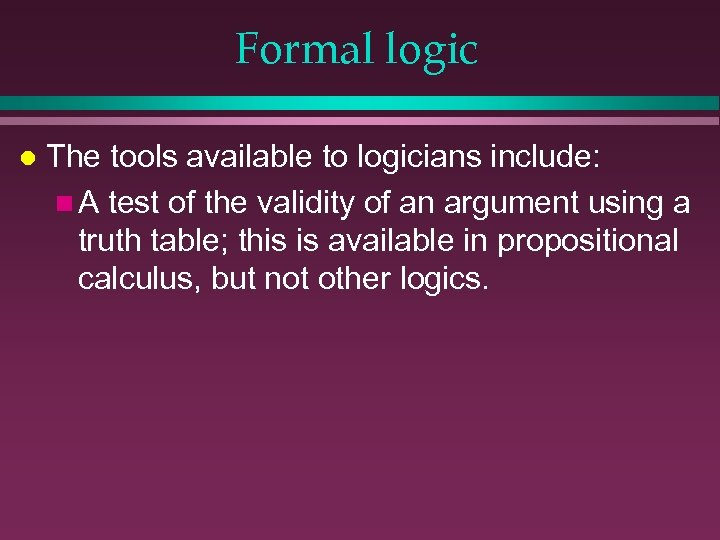Formal logic l The tools available to logicians include: n A test of the validity of an argument using a truth table; this is available in propositional calculus, but not other logics.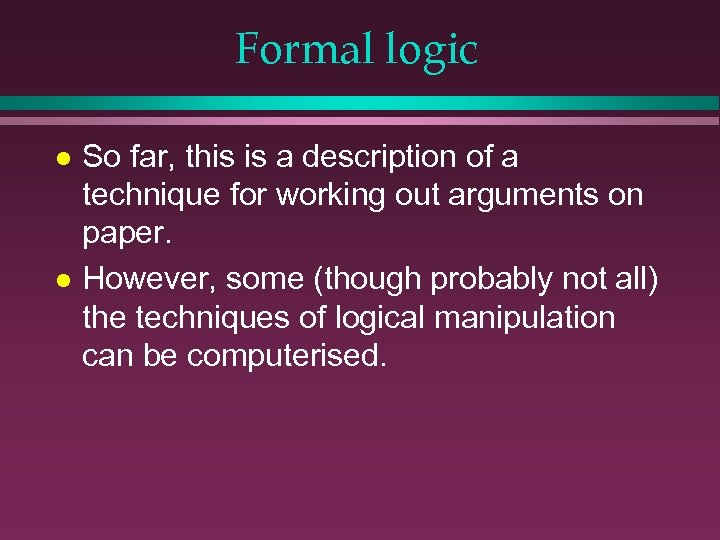Formal logic l l So far, this is a description of a technique for working out arguments on paper. However, some (though probably not all) the techniques of logical manipulation can be computerised.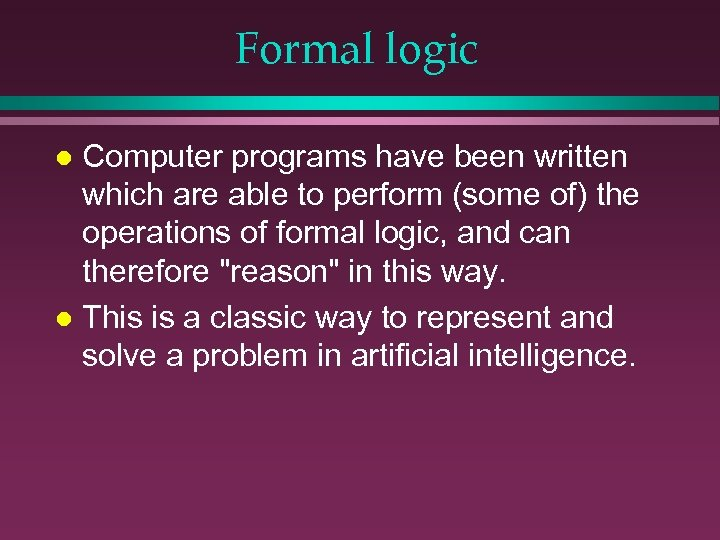Formal logic Computer programs have been written which are able to perform (some of) the operations of formal logic, and can therefore "reason" in this way. l This is a classic way to represent and solve a problem in artificial intelligence. l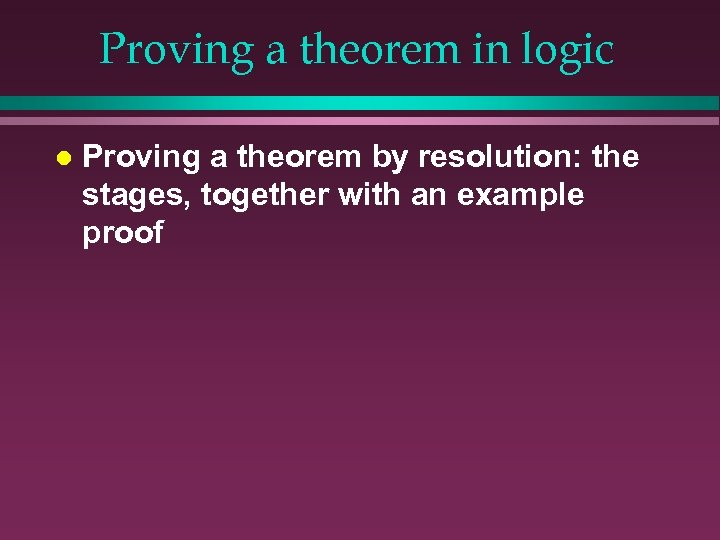Proving a theorem in logic l Proving a theorem by resolution: the stages, together with an example proof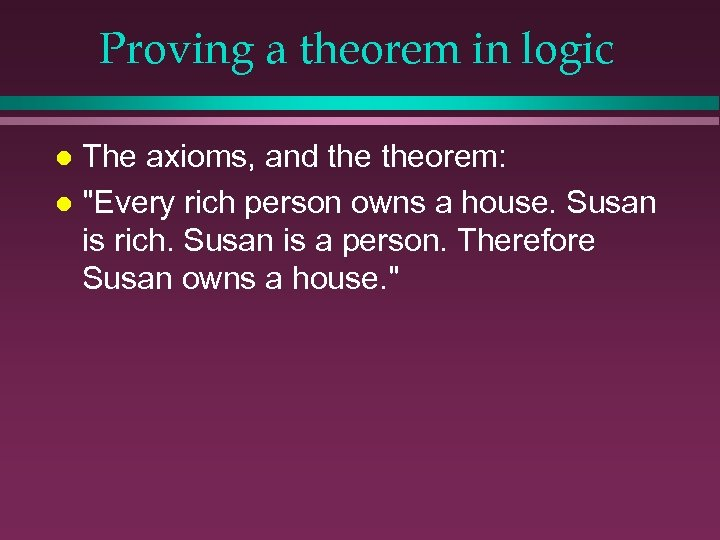Proving a theorem in logic The axioms, and theorem: l "Every rich person owns a house. Susan is rich. Susan is a person. Therefore Susan owns a house. " l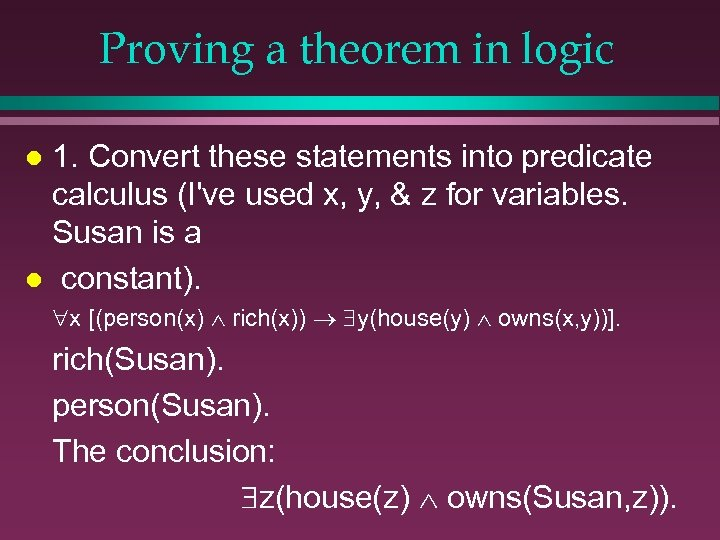Proving a theorem in logic 1. Convert these statements into predicate calculus (I've used x, y, & z for variables. Susan is a l constant). l x [(person(x) rich(x)) y(house(y) owns(x, y))]. rich(Susan). person(Susan). The conclusion: z(house(z) owns(Susan, z)).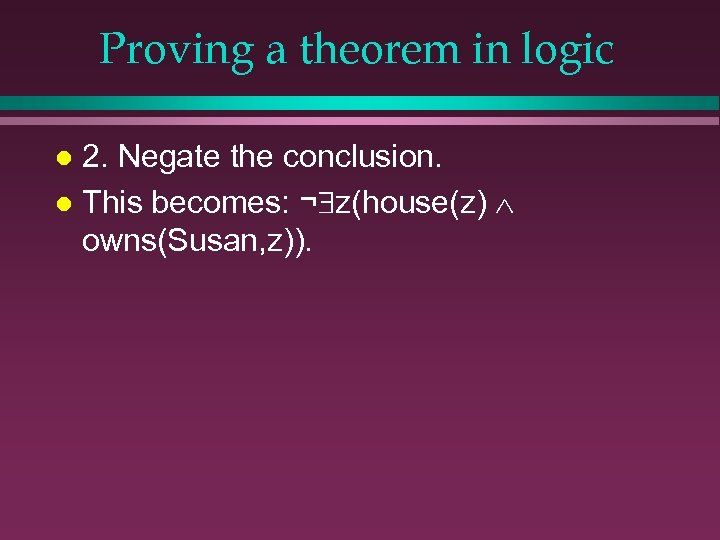Proving a theorem in logic 2. Negate the conclusion. l This becomes: ¬ z(house(z) owns(Susan, z)). l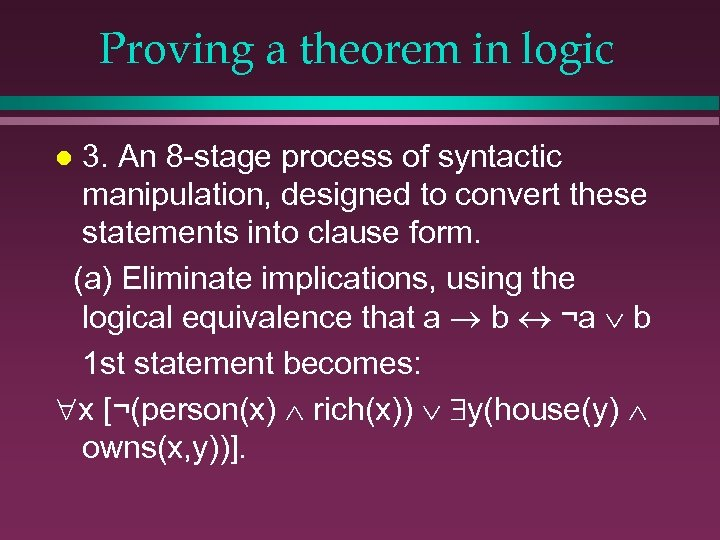Proving a theorem in logic 3. An 8 -stage process of syntactic manipulation, designed to convert these statements into clause form. (a) Eliminate implications, using the logical equivalence that a b ¬a b 1 st statement becomes: x [¬(person(x) rich(x)) y(house(y) owns(x, y))]. l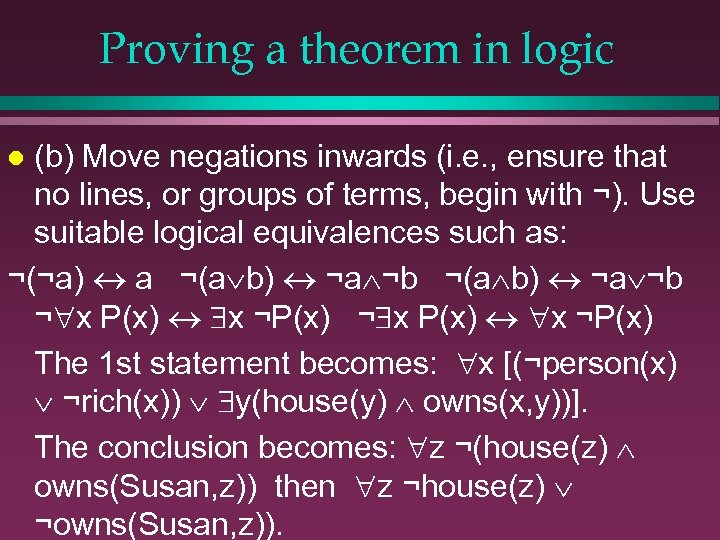Proving a theorem in logic (b) Move negations inwards (i. e. , ensure that no lines, or groups of terms, begin with ¬). Use suitable logical equivalences such as: ¬(¬a) a ¬(a b) ¬a ¬b ¬ x P(x) x ¬P(x) The 1 st statement becomes: x [(¬person(x) ¬rich(x)) y(house(y) owns(x, y))]. The conclusion becomes: z ¬(house(z) owns(Susan, z)) then z ¬house(z) ¬owns(Susan, z)). l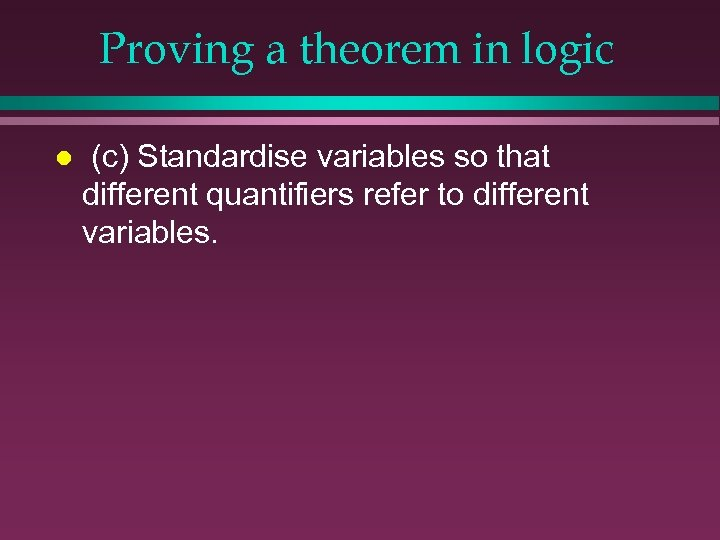Proving a theorem in logic l (c) Standardise variables so that different quantifiers refer to different variables.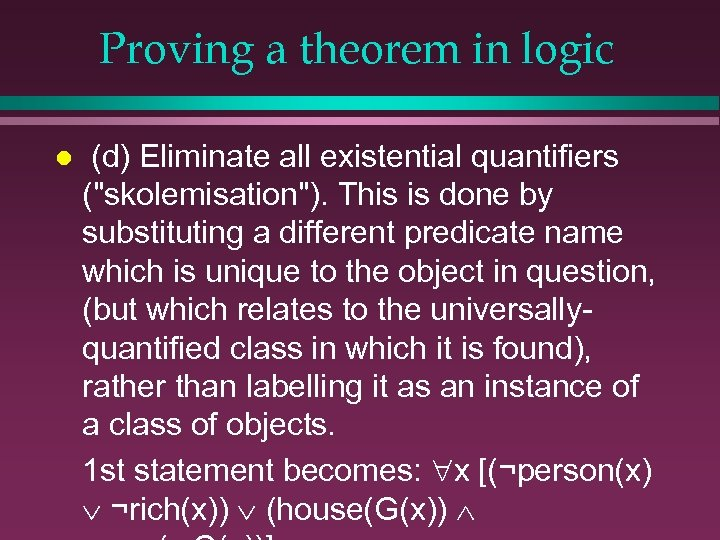Proving a theorem in logic l (d) Eliminate all existential quantifiers ("skolemisation"). This is done by substituting a different predicate name which is unique to the object in question, (but which relates to the universallyquantified class in which it is found), rather than labelling it as an instance of a class of objects. 1 st statement becomes: x [(¬person(x) ¬rich(x)) (house(G(x))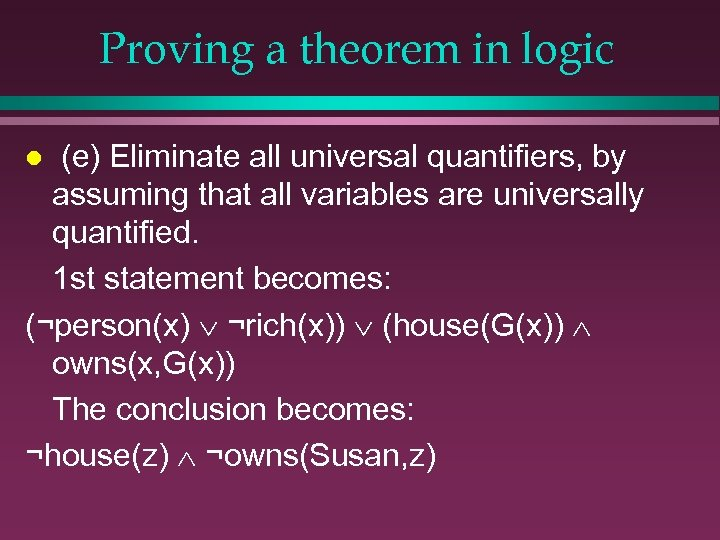Proving a theorem in logic (e) Eliminate all universal quantifiers, by assuming that all variables are universally quantified. 1 st statement becomes: (¬person(x) ¬rich(x)) (house(G(x)) owns(x, G(x)) The conclusion becomes: ¬house(z) ¬owns(Susan, z) l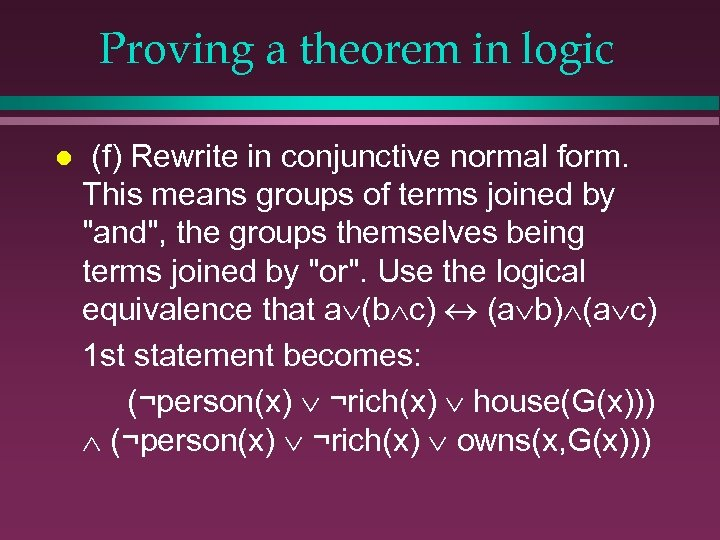Proving a theorem in logic l (f) Rewrite in conjunctive normal form. This means groups of terms joined by "and", the groups themselves being terms joined by "or". Use the logical equivalence that a (b c) (a b) (a c) 1 st statement becomes: (¬person(x) ¬rich(x) house(G(x))) (¬person(x) ¬rich(x) owns(x, G(x)))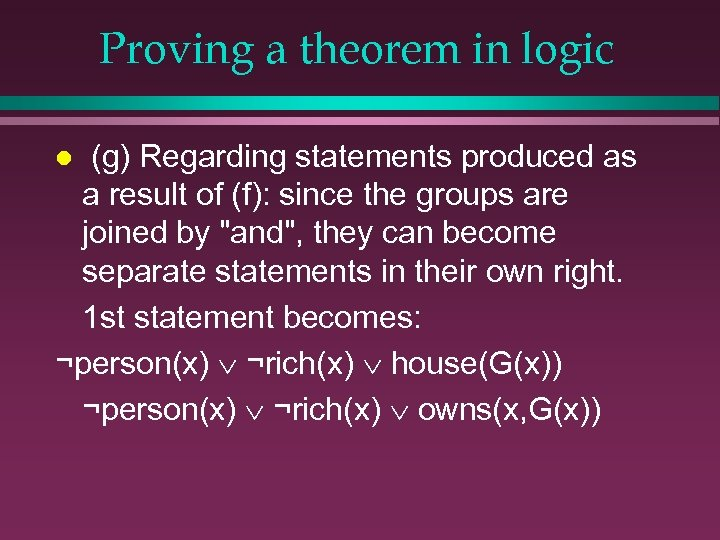Proving a theorem in logic (g) Regarding statements produced as a result of (f): since the groups are joined by "and", they can become separate statements in their own right. 1 st statement becomes: ¬person(x) ¬rich(x) house(G(x)) ¬person(x) ¬rich(x) owns(x, G(x)) l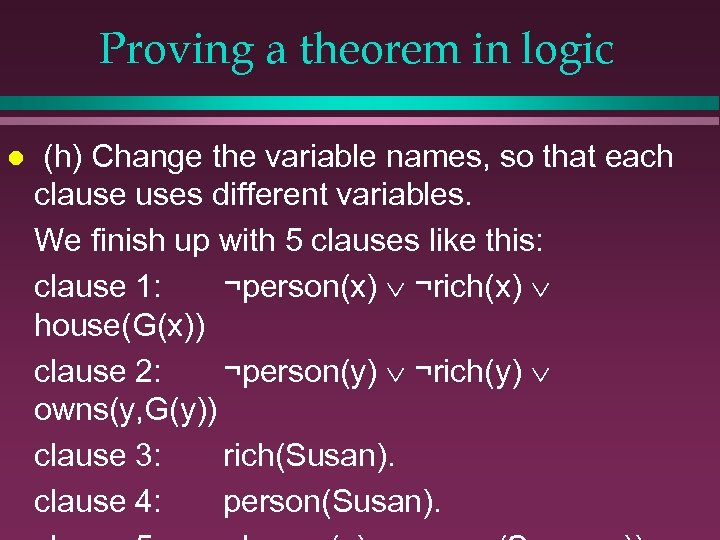Proving a theorem in logic l (h) Change the variable names, so that each clause uses different variables. We finish up with 5 clauses like this: clause 1: ¬person(x) ¬rich(x) house(G(x)) clause 2: ¬person(y) ¬rich(y) owns(y, G(y)) clause 3: rich(Susan). clause 4: person(Susan).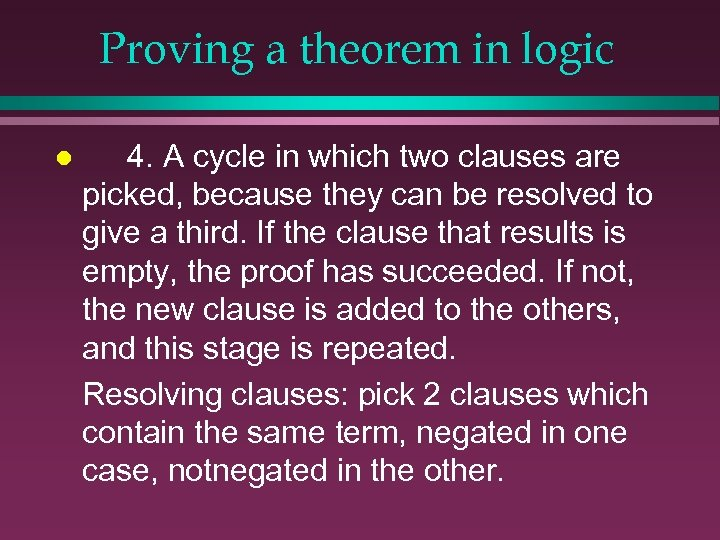Proving a theorem in logic l 4. A cycle in which two clauses are picked, because they can be resolved to give a third. If the clause that results is empty, the proof has succeeded. If not, the new clause is added to the others, and this stage is repeated. Resolving clauses: pick 2 clauses which contain the same term, negated in one case, notnegated in the other.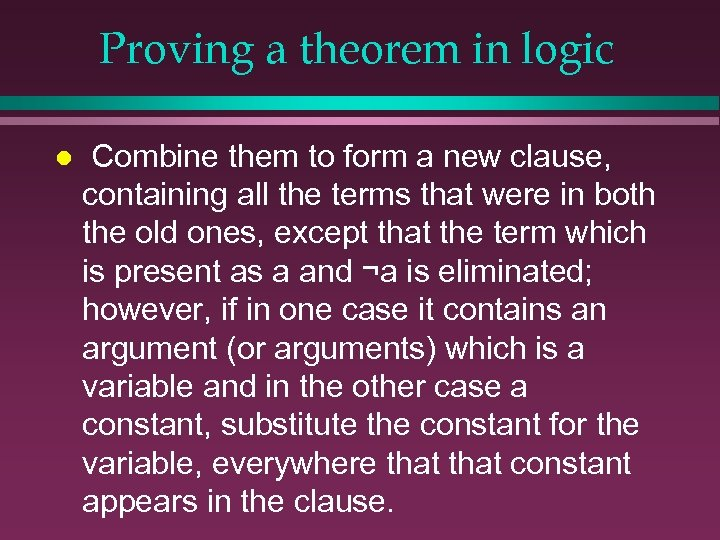Proving a theorem in logic l Combine them to form a new clause, containing all the terms that were in both the old ones, except that the term which is present as a and ¬a is eliminated; however, if in one case it contains an argument (or arguments) which is a variable and in the other case a constant, substitute the constant for the variable, everywhere that constant appears in the clause.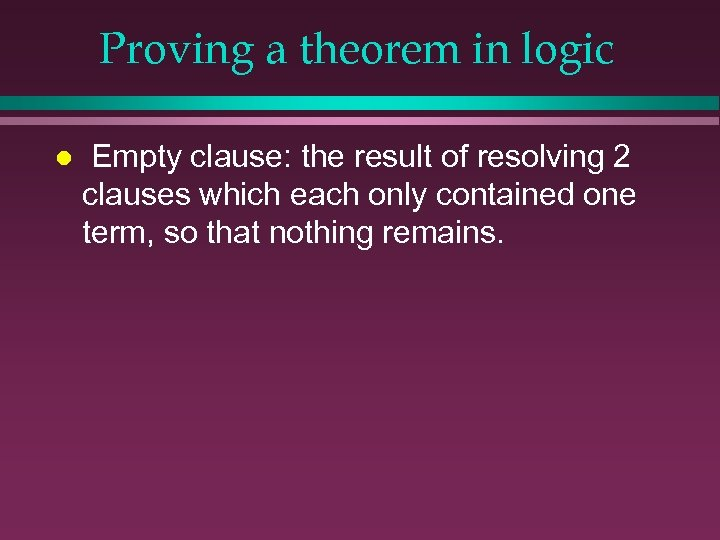Proving a theorem in logic l Empty clause: the result of resolving 2 clauses which each only contained one term, so that nothing remains.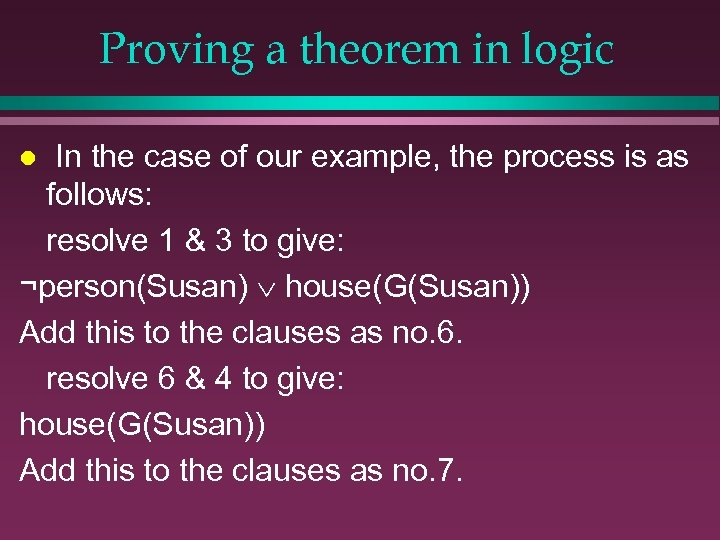Proving a theorem in logic In the case of our example, the process is as follows: resolve 1 & 3 to give: ¬person(Susan) house(G(Susan)) Add this to the clauses as no. 6. resolve 6 & 4 to give: house(G(Susan)) Add this to the clauses as no. 7. l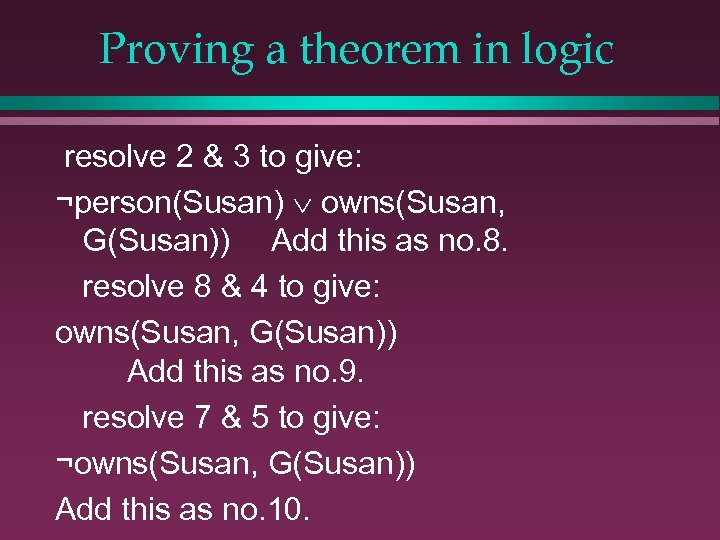Proving a theorem in logic resolve 2 & 3 to give: ¬person(Susan) owns(Susan, G(Susan)) Add this as no. 8. resolve 8 & 4 to give: owns(Susan, G(Susan)) Add this as no. 9. resolve 7 & 5 to give: ¬owns(Susan, G(Susan)) Add this as no. 10.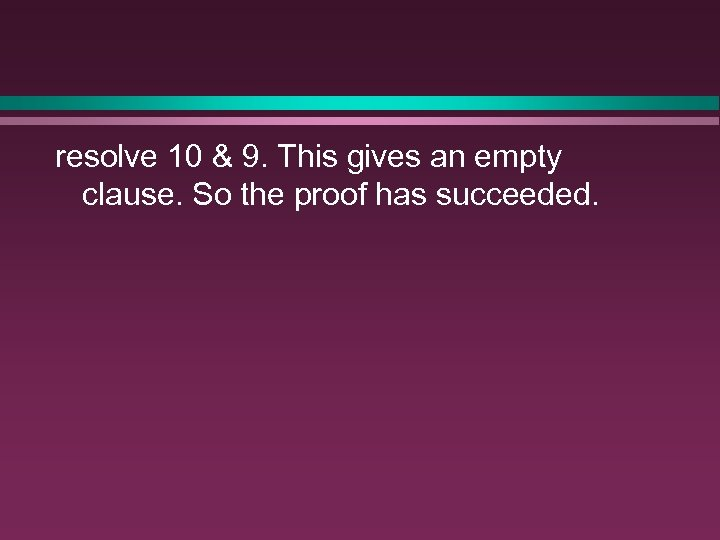resolve 10 & 9. This gives an empty clause. So the proof has succeeded.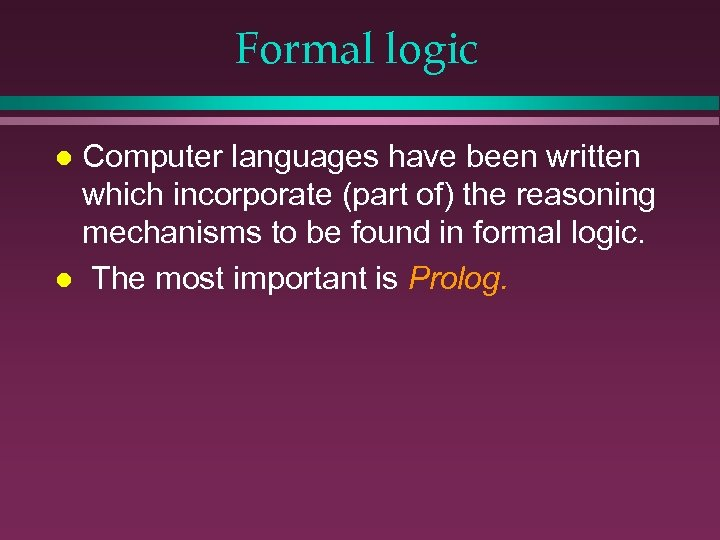Formal logic Computer languages have been written which incorporate (part of) the reasoning mechanisms to be found in formal logic. l The most important is Prolog. l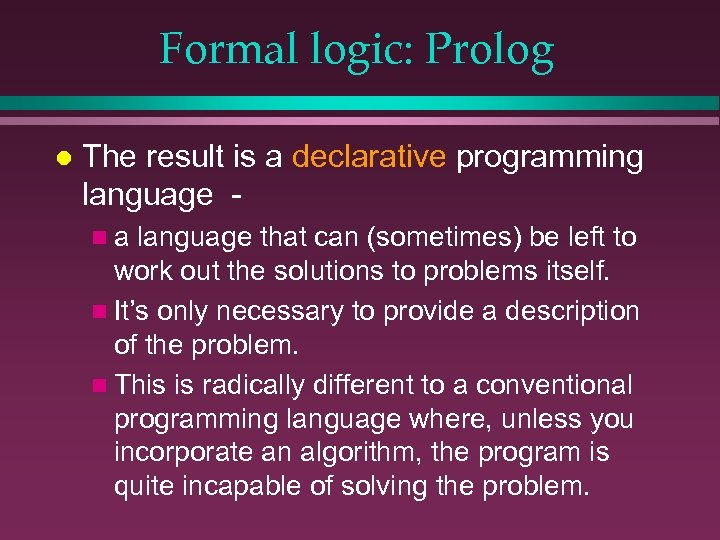Formal logic: Prolog l The result is a declarative programming language na language that can (sometimes) be left to work out the solutions to problems itself. n It’s only necessary to provide a description of the problem. n This is radically different to a conventional programming language where, unless you incorporate an algorithm, the program is quite incapable of solving the problem.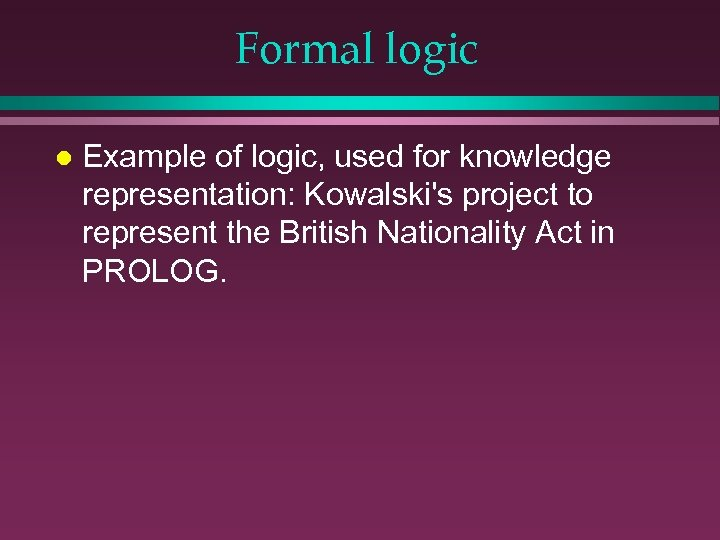Formal logic l Example of logic, used for knowledge representation: Kowalski's project to represent the British Nationality Act in PROLOG.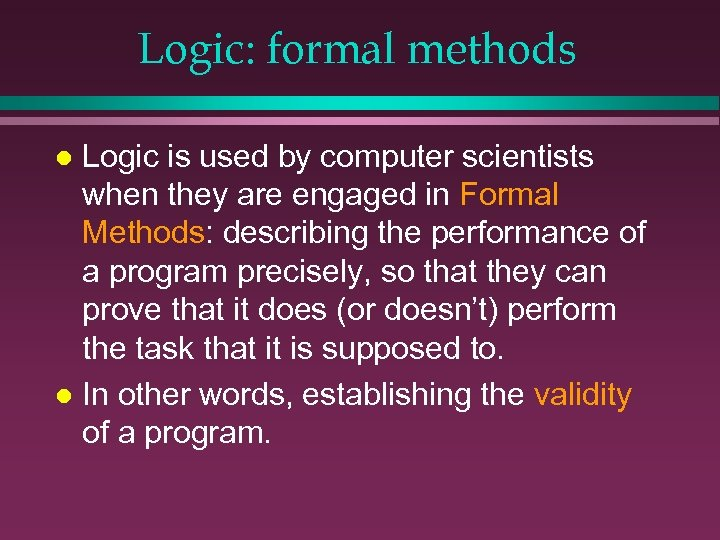Logic: formal methods Logic is used by computer scientists when they are engaged in Formal Methods: describing the performance of a program precisely, so that they can prove that it does (or doesn’t) perform the task that it is supposed to. l In other words, establishing the validity of a program. l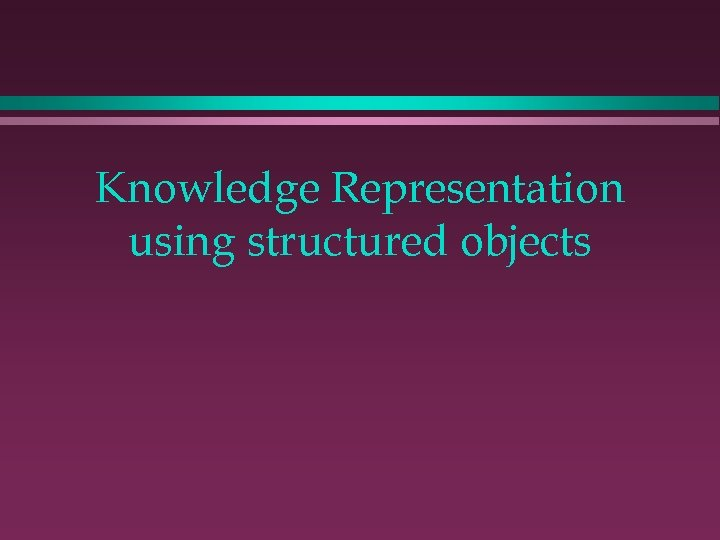Knowledge Representation using structured objects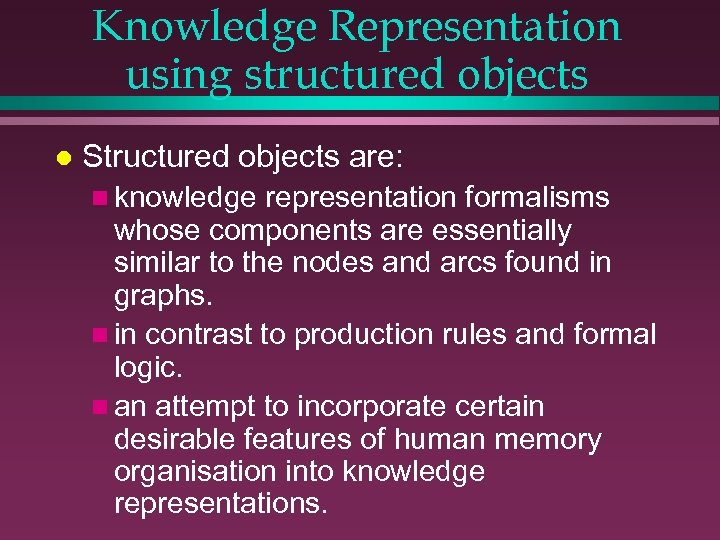Knowledge Representation using structured objects l Structured objects are: n knowledge representation formalisms whose components are essentially similar to the nodes and arcs found in graphs. n in contrast to production rules and formal logic. n an attempt to incorporate certain desirable features of human memory organisation into knowledge representations.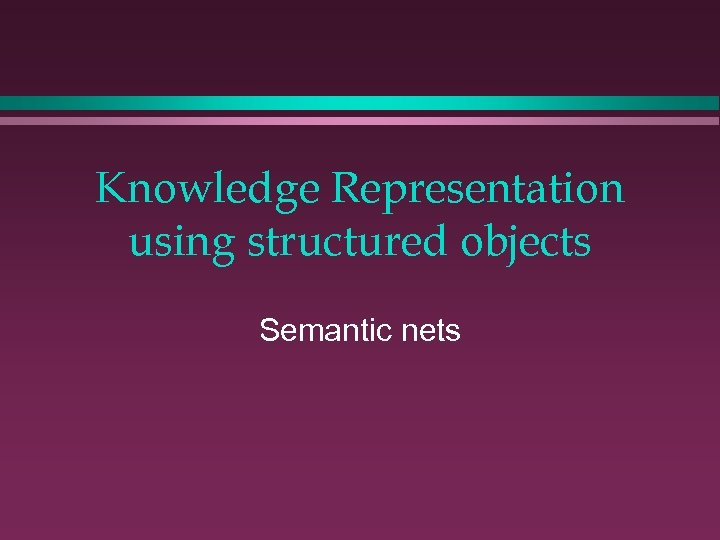Knowledge Representation using structured objects Semantic nets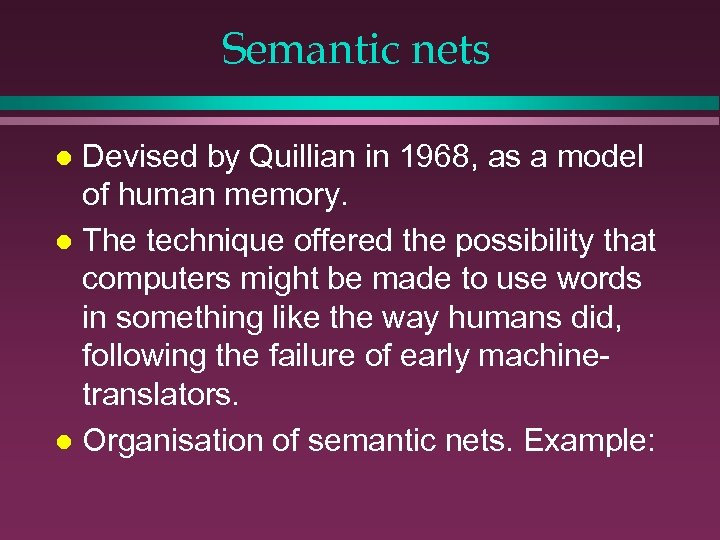Semantic nets Devised by Quillian in 1968, as a model of human memory. l The technique offered the possibility that computers might be made to use words in something like the way humans did, following the failure of early machinetranslators. l Organisation of semantic nets. Example: l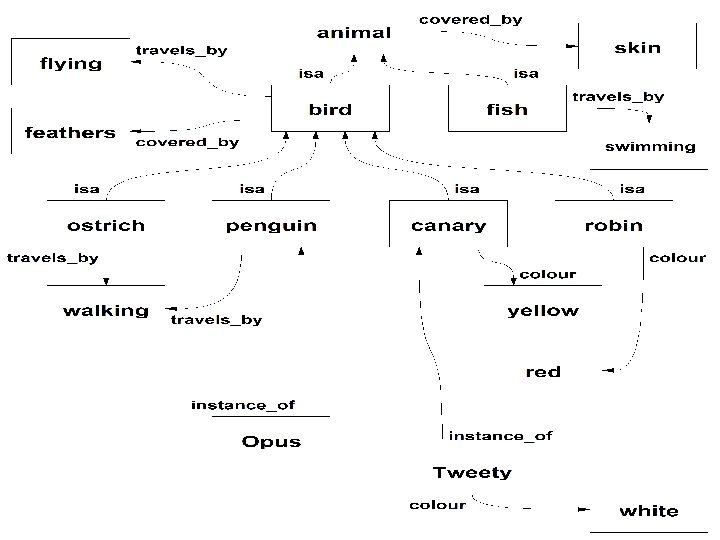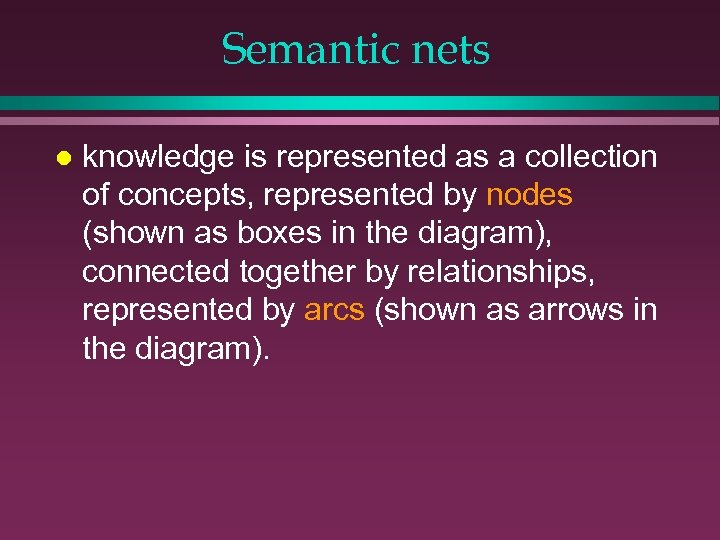Semantic nets l knowledge is represented as a collection of concepts, represented by nodes (shown as boxes in the diagram), connected together by relationships, represented by arcs (shown as arrows in the diagram).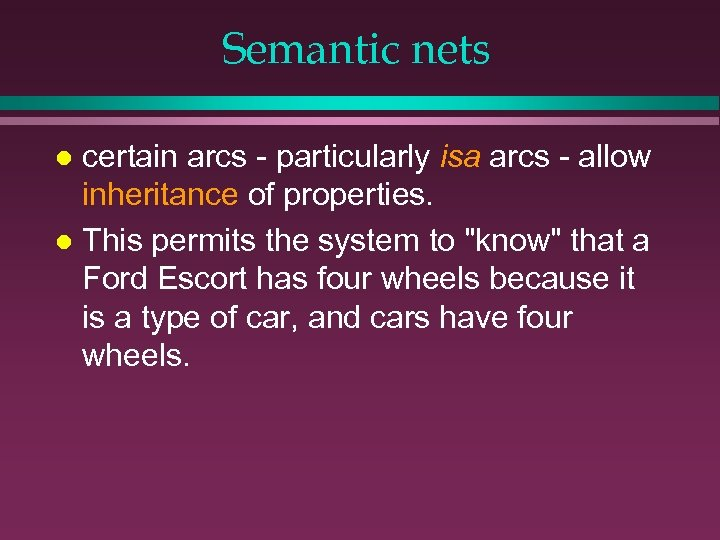Semantic nets certain arcs - particularly isa arcs - allow inheritance of properties. l This permits the system to "know" that a Ford Escort has four wheels because it is a type of car, and cars have four wheels. l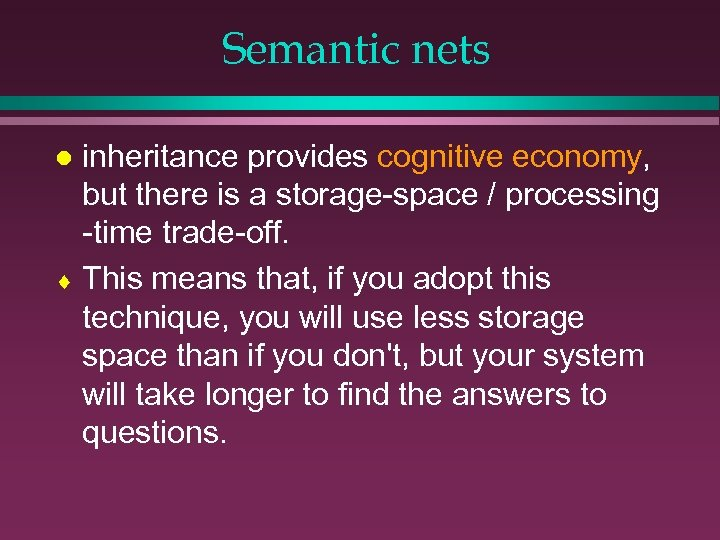Semantic nets inheritance provides cognitive economy, but there is a storage-space / processing -time trade-off. ¨ This means that, if you adopt this technique, you will use less storage space than if you don't, but your system will take longer to find the answers to questions. l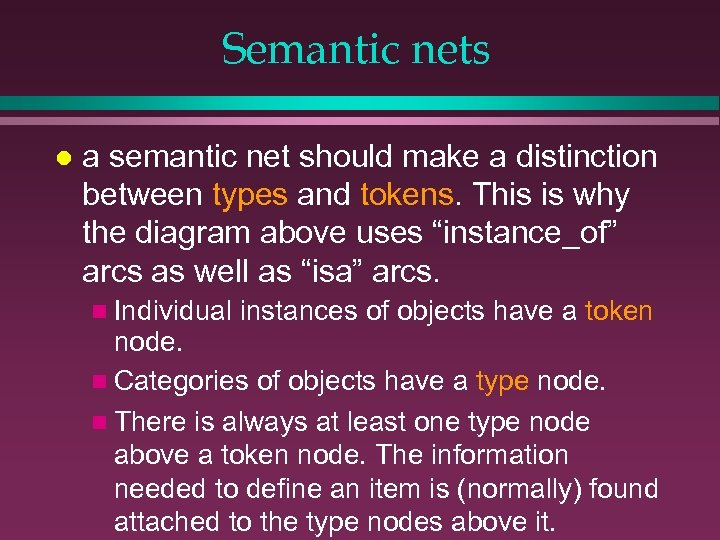Semantic nets l a semantic net should make a distinction between types and tokens. This is why the diagram above uses “instance_of” arcs as well as “isa” arcs. n Individual instances of objects have a token node. n Categories of objects have a type node. n There is always at least one type node above a token node. The information needed to define an item is (normally) found attached to the type nodes above it.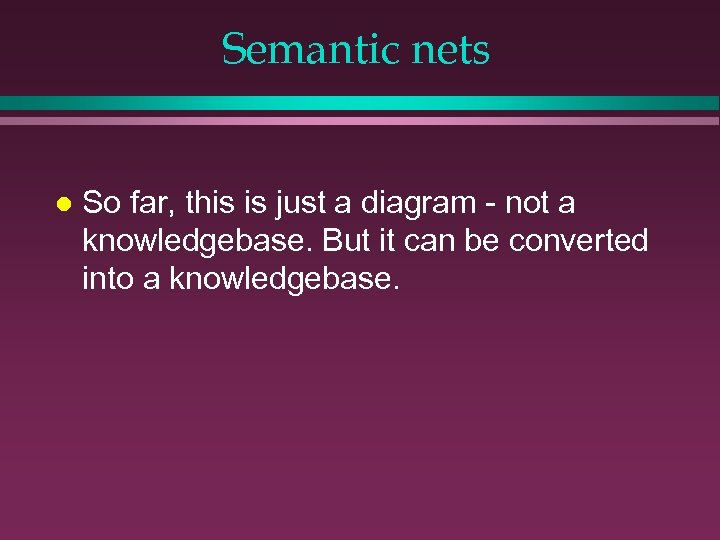Semantic nets l So far, this is just a diagram - not a knowledgebase. But it can be converted into a knowledgebase.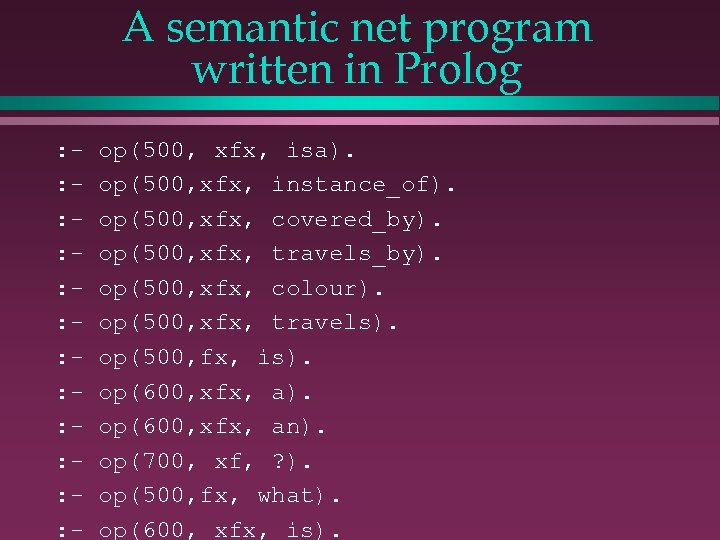A semantic net program written in Prolog : : : - op(500, xfx, isa). op(500, xfx, instance_of). op(500, xfx, covered_by). op(500, xfx, travels_by). op(500, xfx, colour). op(500, xfx, travels). op(500, fx, is). op(600, xfx, an). op(700, xf, ? ). op(500, fx, what). op(600, xfx, is).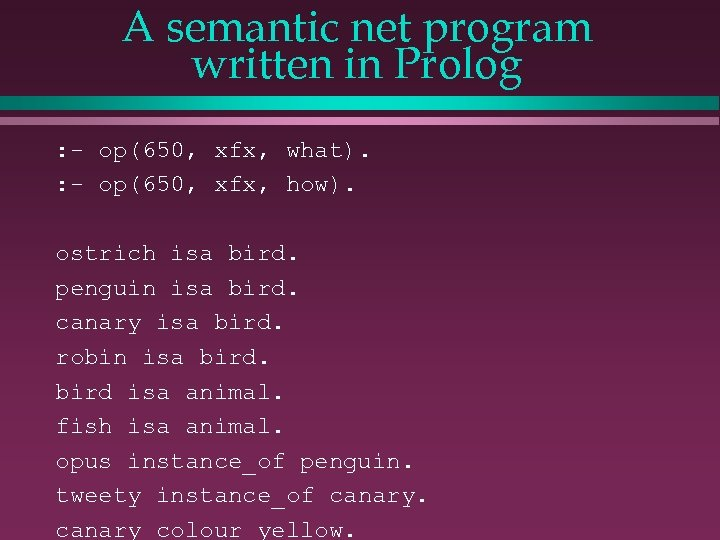A semantic net program written in Prolog : - op(650, xfx, what). : - op(650, xfx, how). ostrich isa bird. penguin isa bird. canary isa bird. robin isa bird isa animal. fish isa animal. opus instance_of penguin. tweety instance_of canary colour yellow.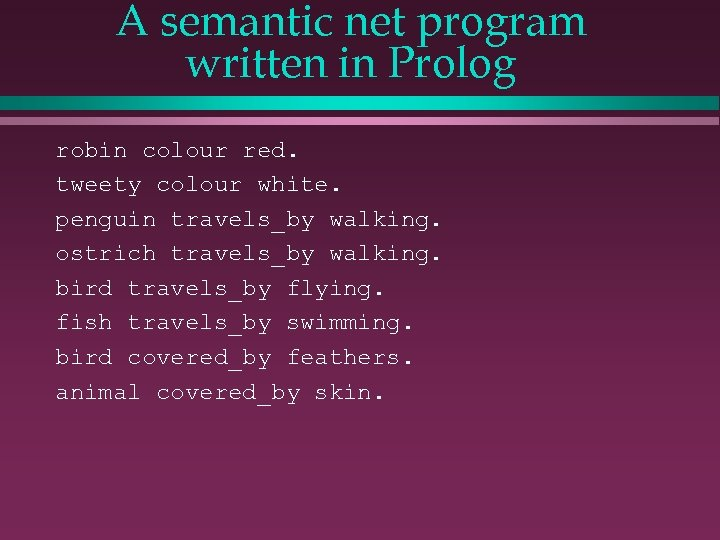A semantic net program written in Prolog robin colour red. tweety colour white. penguin travels_by walking. ostrich travels_by walking. bird travels_by flying. fish travels_by swimming. bird covered_by feathers. animal covered_by skin.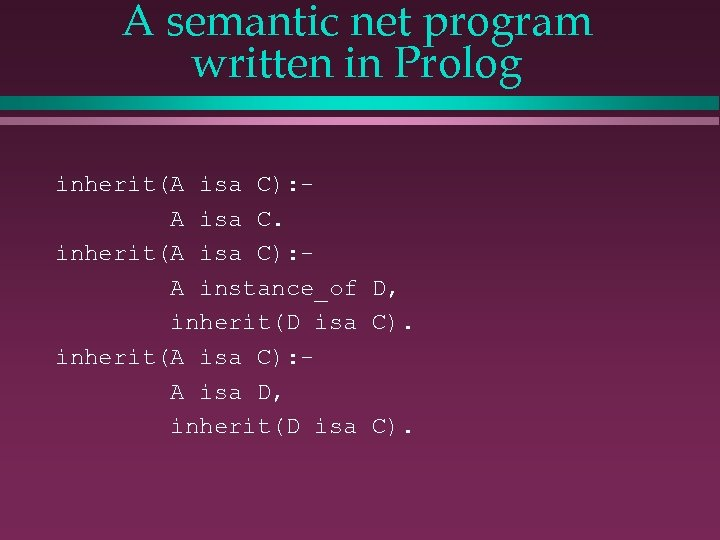A semantic net program written in Prolog inherit(A isa C): A isa C. inherit(A isa C): A instance_of D, inherit(D isa C). inherit(A isa C): A isa D, inherit(D isa C).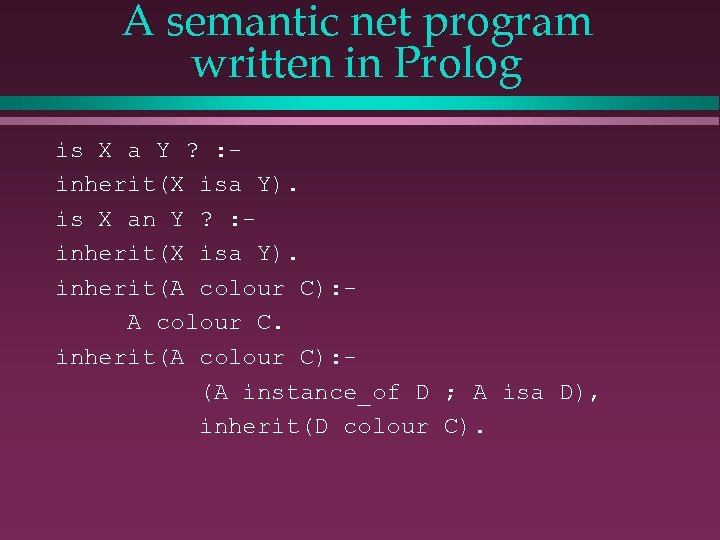A semantic net program written in Prolog is X a Y ? : inherit(X isa Y). is X an Y ? : inherit(X isa Y). inherit(A colour C): A colour C. inherit(A colour C): (A instance_of D ; A isa D), inherit(D colour C).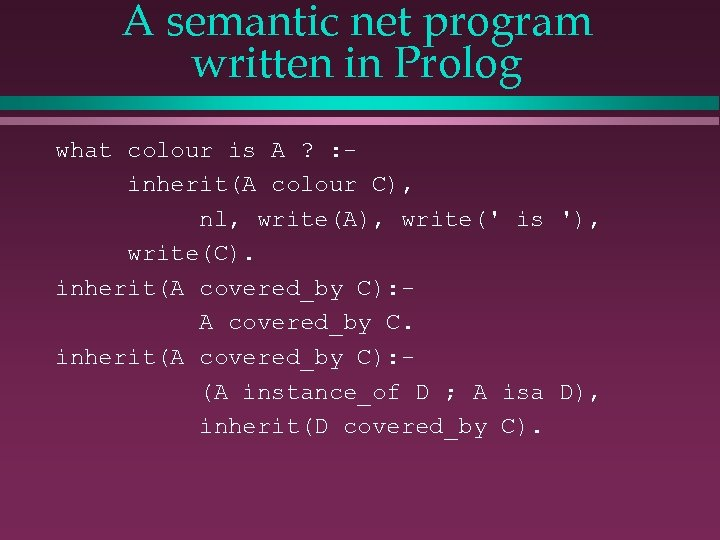A semantic net program written in Prolog what colour is A ? : inherit(A colour C), nl, write(A), write(' is '), write(C). inherit(A covered_by C): A covered_by C. inherit(A covered_by C): (A instance_of D ; A isa D), inherit(D covered_by C).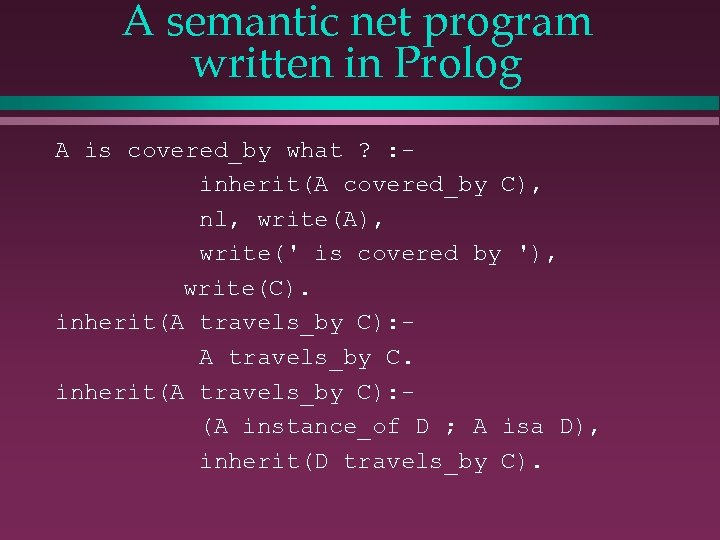A semantic net program written in Prolog A is covered_by what ? : inherit(A covered_by C), nl, write(A), write(' is covered by '), write(C). inherit(A travels_by C): A travels_by C. inherit(A travels_by C): (A instance_of D ; A isa D), inherit(D travels_by C).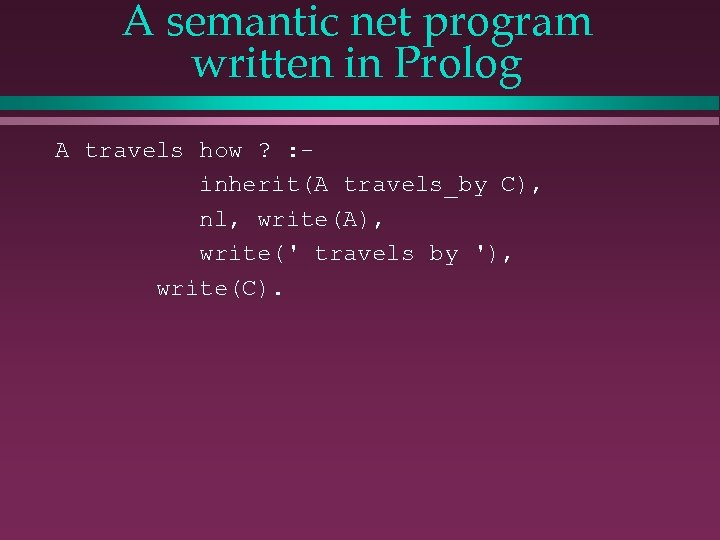A semantic net program written in Prolog A travels how ? : inherit(A travels_by C), nl, write(A), write(' travels by '), write(C).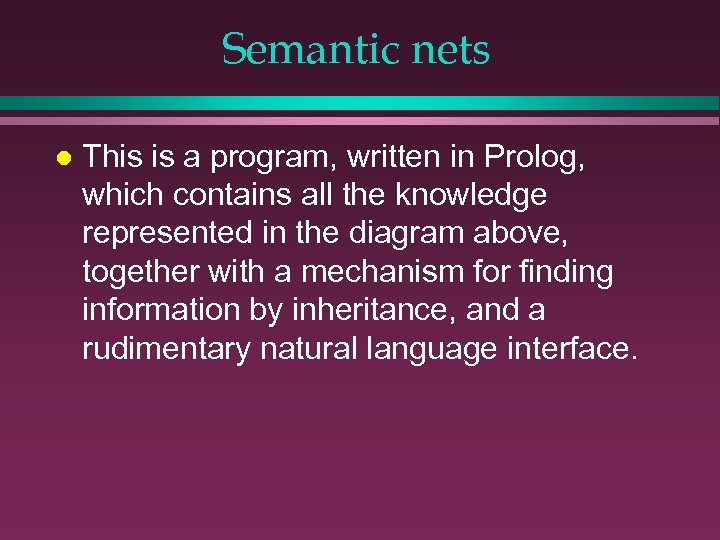Semantic nets l This is a program, written in Prolog, which contains all the knowledge represented in the diagram above, together with a mechanism for finding information by inheritance, and a rudimentary natural language interface.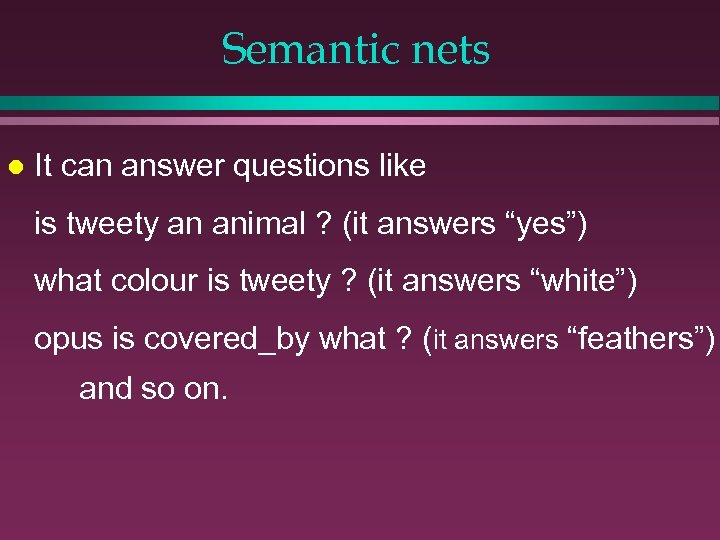Semantic nets l It can answer questions like is tweety an animal ? (it answers “yes”) what colour is tweety ? (it answers “white”) opus is covered_by what ? (it answers “feathers”) and so on.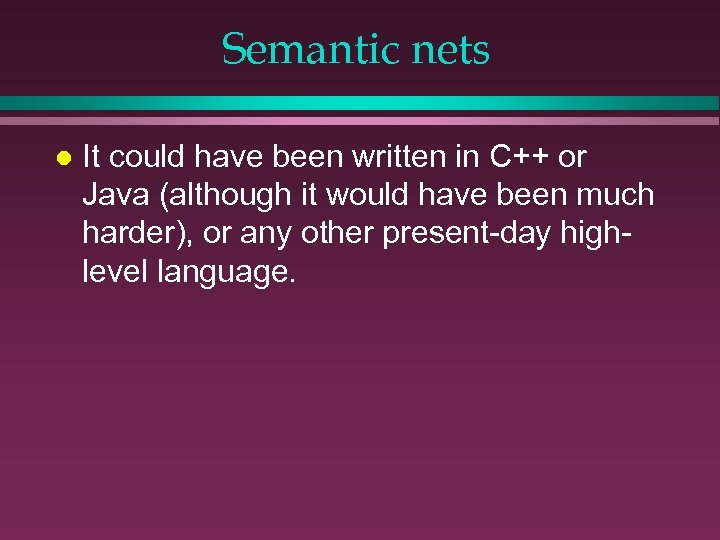Semantic nets l It could have been written in C++ or Java (although it would have been much harder), or any other present-day highlevel language.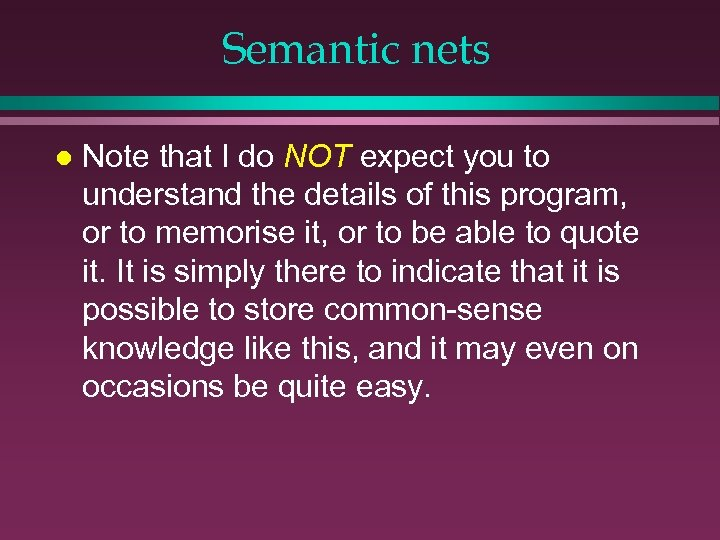Semantic nets l Note that I do NOT expect you to understand the details of this program, or to memorise it, or to be able to quote it. It is simply there to indicate that it is possible to store common-sense knowledge like this, and it may even on occasions be quite easy.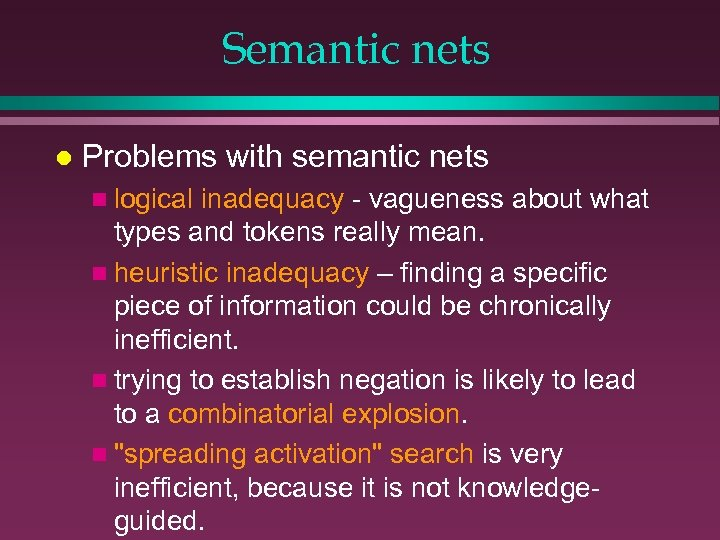Semantic nets l Problems with semantic nets n logical inadequacy - vagueness about what types and tokens really mean. n heuristic inadequacy – finding a specific piece of information could be chronically inefficient. n trying to establish negation is likely to lead to a combinatorial explosion. n "spreading activation" search is very inefficient, because it is not knowledgeguided.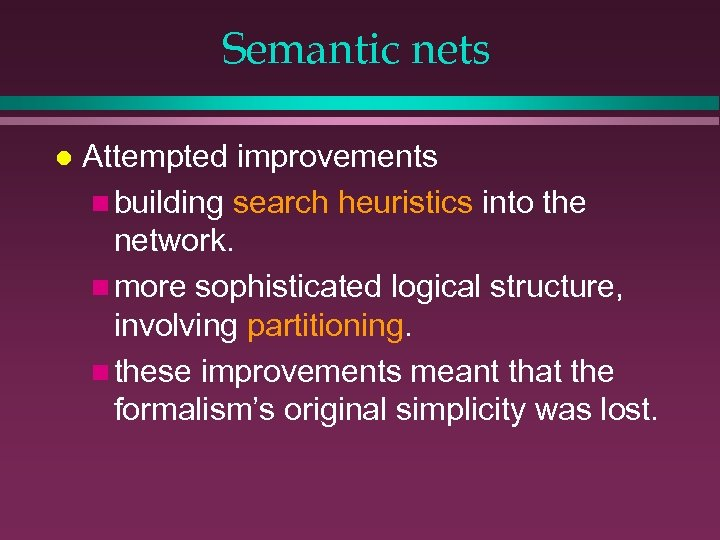Semantic nets l Attempted improvements n building search heuristics into the network. n more sophisticated logical structure, involving partitioning. n these improvements meant that the formalism’s original simplicity was lost.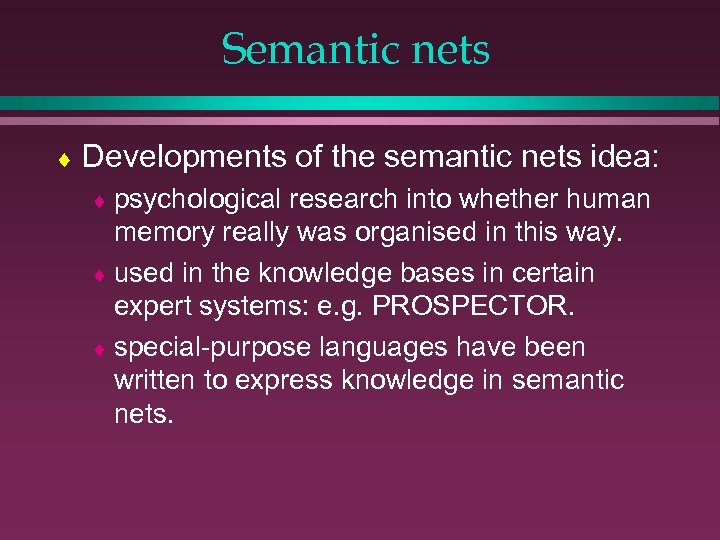Semantic nets ¨ Developments of the semantic nets idea: ¨ psychological research into whether human memory really was organised in this way. ¨ used in the knowledge bases in certain expert systems: e. g. PROSPECTOR. ¨ special-purpose languages have been written to express knowledge in semantic nets.